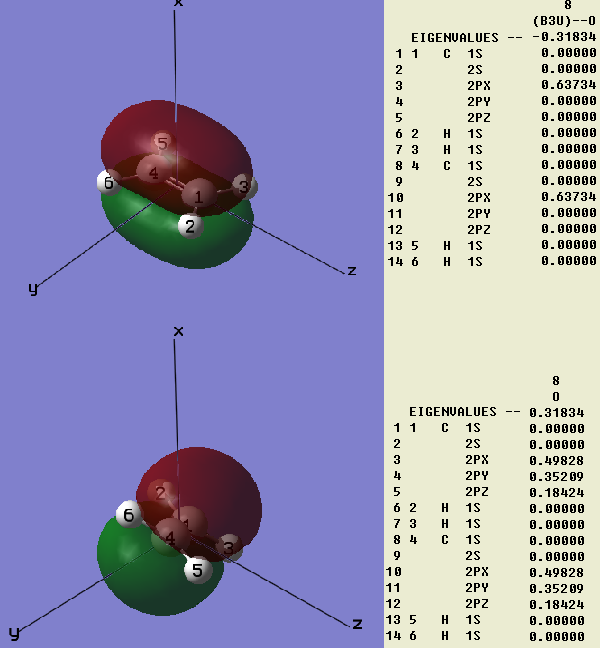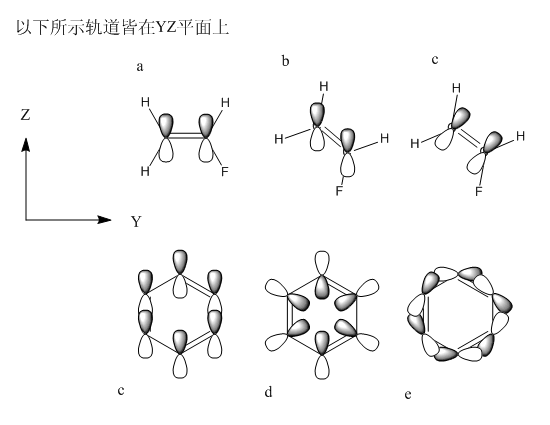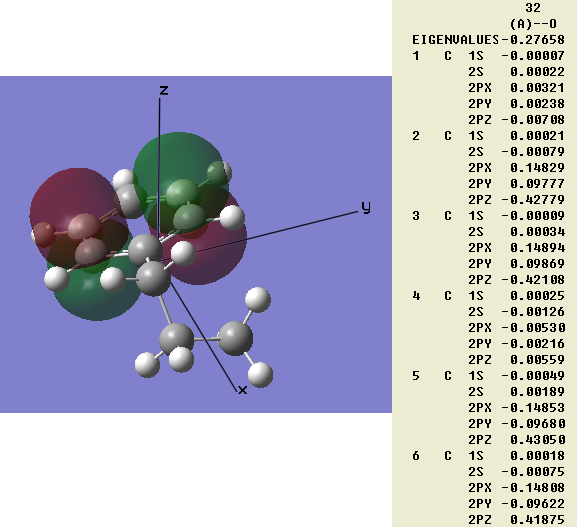# 谈谈原子轨道朝向引起的量化计算结果与经验观念的差异(X'FX)(i,j)=∑[k]X'(i,k)(FX)(k,j)=∑[k]X(k,i)"(FX)(k,j)=∑[k]X(k,i)"∑[l]F(k,l)X(l,j)=∑[k]∑[l]X(k,i)"X(l,j)<a(k)|f|a(l)>=<∑[k]X(k,i)a(k)|f|∑[l]X(l,j)a(l)>=<b(i)|f|b(j)>=F`(i,j)

（半经验方法常使用ZDO近似，因而重叠矩阵往往近似为单位矩阵，Fock矩阵中很多多中心双电子积分项都成为了0，假设称为矩阵K。此时方法不再具有基函数线性变换不变性，这与前面讨论的HF方程的情况不同。一方面是S=I的近似明显使上述推导不成立，因为不同基函数系下S显然不同；另一方面，半经验方法出于数值求解方便，根据ZDO近似对F进行不同程度的篡改，得到的K并不对应于某个算符，故K与K`不是同一个算符在不同表象下的矩阵形式，上述线性变换不变性的证明也不成立。但满足线性变换不变性是重要的，尤其是要满足其中同原子上基函数的线性变换不变性，否则将失去物理意义，所以很多半经验方法通过将双电子积分用预设参数取代，不仅加速了计算，也同时满足了这个要求。比如使双电子积分值不看轨道具体类型，只取决于所在中心，则杂化、旋转引起的同原子上的基函数混合对结果将不产生影响。）